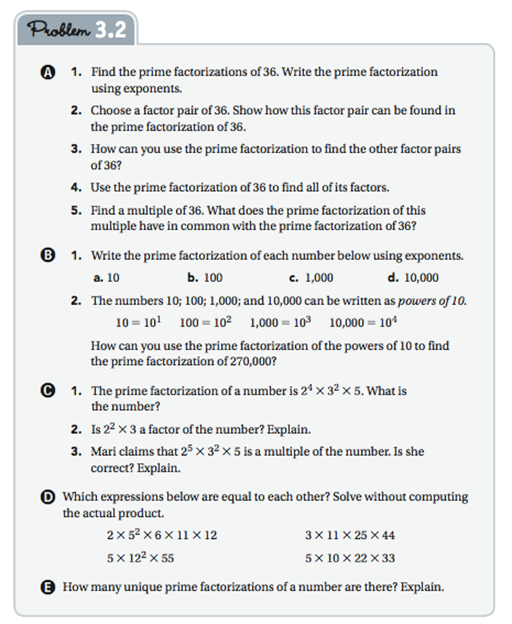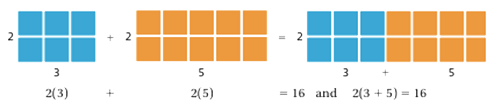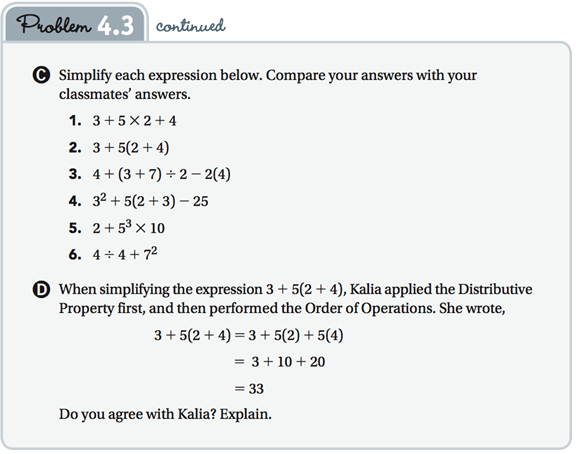Display Accessibility Tools

# Prime Time and the CCSSM Algebra

This document compliments the paper, CCSSM Expressions and Equations in CMP3 Grade 6, and provides guidance for instances where students engage with the Algebra ideas in Prime Time.

# CCSS.MATH.CONTENT.6.EE.A.1

Write and evaluate numerical expressions involving whole-number exponents.

## Prime Time Problem 3.2

Exponential notation is introduced in Prime Time Investigation 3, where students’ exploration of factorization strings in Problem 3.1 leads to the introduction of prime factorization strings and exponential notation in Problem 3.2.# CCSS.MATH.CONTENT.6.EE.A.2.A

Write expressions that record operations with numbers and with letters standing for numbers. For example, express the calculation "Subtract y from 5" as 5 - y.

## Prime Time Problem 4.4 Choosing an Operation

Writing numeric and symbolic expressions and sentences to represent the operations required to solve problems is one of the most important skills in applied mathematics. Each unit of CMP3 includes many activities that develop understanding and proficiency in work on such modeling tasks.

For instance, Problem 4.4 of Prime Time asks students to analyze five different word problem situations, to decide which operations are needed to solve the problems, and to write one or more expressions that represent those operations. For example:

Dan is selling fudge to raise money for the school band. Each box of fudge costs \$8. One week he sells 15 boxes, and the next week he sells 17 boxes. How much money has Dan made at the end of the two weeks?

These exercises that involve writing expressions to record problem-solving operations result in number sentences like:

8 x (15 + 17)
or equivalenty
8 x 15 + 8 x 17

# CCSS.MATH.CONTENT.6.EE.A.2.B

Identify parts of an expression using mathematical terms (sum, term, product, factor, quotient, coefficient); view one or more parts of an expression as a single entity. For example, describe the expression 2 (8 + 7) as a product of two factors; view (8 + 7) as both a single entity and a sum of two terms.

## Prime Time Problem 4.2

The mathematical vocabulary of sum, product, and factor are introduced early in the first sixth grade unit Prime Time and are used throughout the 6th grade CMP units.  In Problem 4.2, the discussion of the Distributive Property and associated mathematical models and symbols are used to help students understand the mathematical meaning of term.  For example:

Lila made the conjecture that the sum of two even numbers is an even number. She used square tiles to show why the sum of two even numbers is always even.Alex wondered of this means that 2(3) + 2(5) = 2(3 + 5).

What do you think? Are Lila and Alex correct? Explain why or why not?

Lila's picture represents an important property of numbers called the Distributive Property. The Distributive Property connects the operations of addition and multiplication.

2(3) + 2(5)       =     2(3 + 5) = 16
sum of two terms    product of two factors

You can write the number 16 as a sum of two quantities, 6 and 10. You can also write the number 16 as the sum of two other quantities, 2(3) and 2(5).

16 = 6 + 10 = 2(3) + 2(5)

You can write the number 16 as a product of two factors, 2 and 8. You can also write 16 as the product of two other factors, 2 and (3 + 5).

16 = 2(8) = 2(3 + 5)

The expressions 2(3) + 2(5) and 2(3 + 5) are equivalent expressions.

2(3) + 2(5) = 2(3 + 5)

# CCSS.MATH.CONTENT.6.EE.A.2.C

Evaluate expressions at specific values of their variables. Include expressions that arise from formulas used in real-world problems. Perform arithmetic operations, including those involving whole-number exponents, in the conventional order when there are no parentheses to specify a particular order (Order of Operations). For example, use the formulas V = s3 and A = 6 s2 to find the volume and surface area of a cube with sides of length s = 1/2.# CCSS.MATH.CONTENT.6.EE.A.3

Apply the properties of operations to generate equivalent expressions. For example, apply the distributive property to the expression 3 (2 + x) to produce the equivalent expression 6 + 3x; apply the distributive property to the expression 24x + 18y to produce the equivalent expression 6 (4x + 3y); apply properties of operations to y + y + y to produce the equivalent expression 3y.

## Prime Time Problems 4.2, 4.3, and 4.4

The fundamental concept of equivalent expressions is a big idea throughout the CMP curriculum. It is introduced in Investigation 4 of Prime Time where students focus on application of the distributive property of multiplication over addition to generate equivalent numeric expressions.

For example, in Prime Time Problem 4.2:

Each of the following numerical expressions represents the area of a rectangle that has been divided into two smaller rectangular pieces. For each expression,

• sketch a rectangle whose area can be represented by the expression.
• write an equivalent expression for the area of the original rectangle.
1. 6(5 + 9)
2. 4(7) + 4(3)

In Prime Time Problem 4.3, students explore the Distributive Property and the Order of Operations, and the common misconception that you must always simplify within the parenthesis before multiplying.

When simplifying the expression 3 + 5(2 + 4), Kalia applied the Distributive Property first, and then performed the Order of Operations. She wrote,

3 + 5(2 + 4) = 3 + 5(2) + 5(4)
= 3 + 10 + 2
= 3 + 10 + 20
= 33

Do you agree with Kalia? Explain.

In Prime Time Problem 4.4, when students “write one or two expressions to represent each” of the 5 problems, they will see equivalent expressions that provide a correct answer for each problem and use the Distributive Property to reason about why the two expressions are equivalent.

For each of the following situations,

• decide wich operations are needed to solve the problem.
• write one or two expressions to represent each problem.
• use the Order of Operations to simplify your expressions.

Dan is selling fudge to raise money for the school band. Each box of fudge costs \$8. One week he sells 15 boxes, and the next week he sells 17 boxes. How much money has Dan made at the end of the two weeks?

# CCSS.MATH.CONTENT.6.EE.A.4

Identify when two expressions are equivalent (i.e., when the two expressions name the same number regardless of which value is substituted into them). For example, the expressions y + y + y and 3y are equivalent because they name the same number regardless of which number y stands for.

## Prime Time Problem 4.3

The idea that equivalence means two expressions give the same outputs is first highlighted in Investigation 4 of Prime Time. Featuring applications of the distributive property, the most common principle for generating equivalent expressions, Problem 4.3 of that investigation states the property in generality with letter names for variables a(b + c) = a(b) + a(c).

# CCSS.MATH.CONTENT.6.EE.B.5

Understand solving an equation or inequality as a process of answering a question: which values from a specified set, if any, make the equation or inequality true? Use substitution to determine whether a given number in a specified set makes an equation or inequality true.

## Prime Time Problem 2.2 D

Solving inequalities does not occur until the Variables and Patterns unit; however, paying attention to those problems that highlight the ideas of inequalities will prepare students to tackle those problems at a later time. Problem 2.2 D in Prime Time is one of those problems that serves multiple purposes, one of which is reasoning quantitatively about inequalities.

Stephan's grandfather told him about how cicadas sometimes damage his orchard. One year, there were so many cicadas, they wrecked the buds on all of his young trees. Both the 13-year old and 17-year cicadas came up that year.

• A. Suppose 13-year and 17-year cicadas both appear this year. When will both types of cicadas next appear together? Explain.
• B. Suppose there are 12-year, 14-year, and 16-year cicadas that all appear this year. After how many years will all three types of cicadas appear together again? Explain.
• C. Stephan developed a method to determine the next time two types of cicadas will appear together. He says that if you multiply the cycles together, you get the next time that both types will appear together. Does Stephan's method work for any pair of cycles? If so, explain why. If not, provide an example for which Stephan's method does not work.
• D. For Questions A and B, decide whether the answer is less than, greater than, or equal to the product of the cicada cycles.

## Prime Time Problem 4.3, associated ACE #24, 25, 26, 27, and 77, 78, 79

Students are engaged in solving equations many times in grade 6 CMP3 units.  For example, application and connection exercises in Investigation 4 of Prime Time ask students to “replace m with a whole number to make 7(4 + m) = 49 true” and “find whole number values of n so that 3(n + 2) is a multiple of 5.”  The intent of these problems is for students to informally reason using what they know of the order of operations and the distributive property to find the values of the variable that make the statements true.

## Summary Table of Algebraic thinking in Prime Time

Prime Time Problem CCSSM Equations and Expressions Standards
Problem 2.2 D CCSS.MATH.CONTENT.6.EE.B.5
Problem 3.2 CCSS.MATH.CONTENT.6.EE.A.1/td>
Problem 4.2 CCSS.MATH.CONTENT.6.EE.A.2.B
CCSS.MATH.CONTENT.6.EE.A.3
Problem 4.3 CCSS.MATH.CONTENT.6.EE.A.2.C
CCSS.MATH.CONTENT.6.EE.A.3
CCSS.MATH.CONTENT.6.EE.A.4
Problem 4.3
ACE #24, 25, 26, 27,and 77, 78, 79
CCSS.MATH.CONTENT.6.EE.B.5
Problem 4.4 CCSS.MATH.CONTENT.6.EE.A.2.A
CCSS.MATH.CONTENT.6.EE.A.3